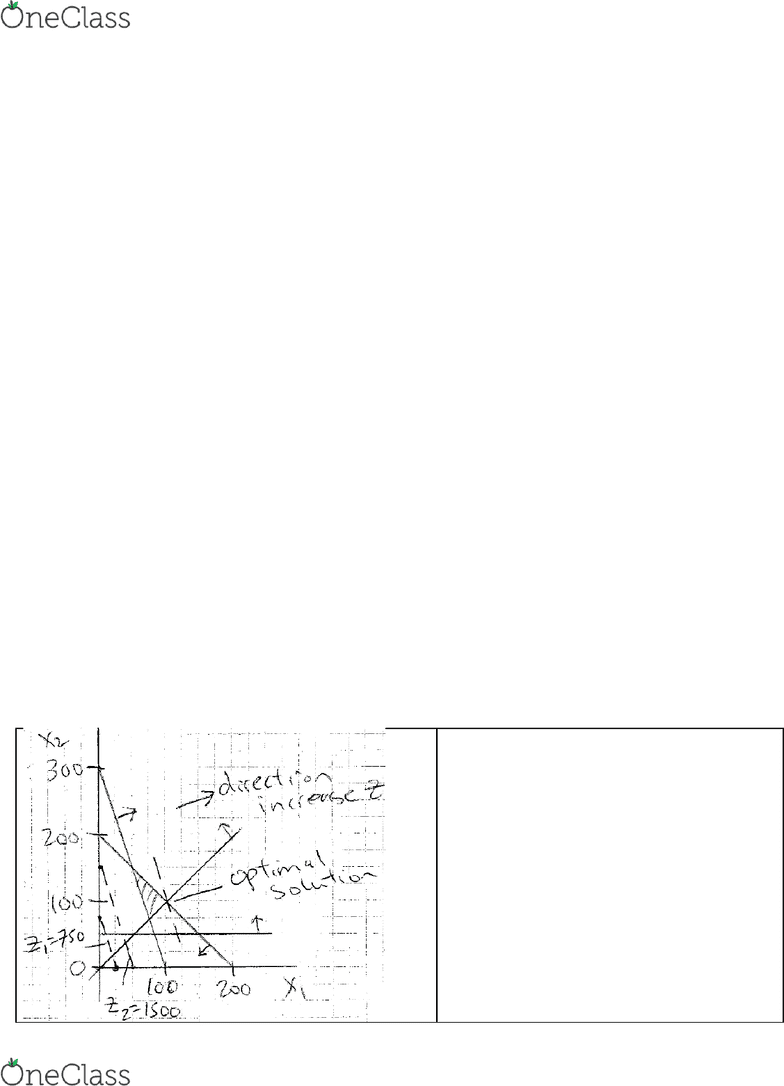Study Guides (390,000)
CA (150,000)
UTSC (10,000)
Midterm

# MGOC10H3 Midterm: MGOC10-MT-SOL-post

Department
Management
Course Code
MGOC10H3
Professor
Vinh Quan
Study Guide
Midterm

This preview shows half of the first page. to view the full 3 pages of the document.Department of Management, UTSC
MGOC10 Analysis for Decision Making – L01/L02 – Midterm Solution
Q1.
(a) a = 0, b = 10000, c = 6000, d = 0
(b)
6– 6 ≤ range coeff x5 ≤ 6 + ∞ so 0 ≤ range coeff x5 ≤ ∞
New Z = old Z – reduction due to coefficient of x5 dropping
= 150000 - (6 - 4)*(x5) = 150000 - (6 - 4)*(5000) =140000
(c)
10000 -5000 ≤ RHS R5 ≤ 10000 +333 get 5000 ≤ RHS R1 ≤ 10333
Dual price R5 = -12 means for each unit increase in RHS, Z worst off by 12.
Worst off means Z goes up. So new Z=150000 + 12(10200 - 10000) = 152400
Q2.
P1, P2 = the number of model 1,2 motors to produce
AA = the number of hours allocated to area A
AB = the number of hours allocated to area B
AC = the number of hours allocated to area C
TBA = the number of hours transferred from B to A
TCA = the number of hours transferred from C to A
TCB = the number of hours transferred from C to B
OTA = OT hours used in area A OTB = OT hours used in area B OTC = OT hours used in area C
Min 80P1 + 65P2 + 55OTA + 55OTB + 55OTC
s.t. 15P1 + 3P2 AA
4P1 + 10P2 AB
4P1 + 8P2 AC
AA = 10000 + OTA + TBA + TCA
AB = 10000 + OTB + TCB - TBA
AC = 18000 + OTC - TCA - TCB
TBA 12000
TCA + TCB 20000
P1 + P2 ≥ 50,000
4P1 ≤ P2
OTA ≤ 5000, OTB ≤ 6000, OTC ≤ 7000
all variables 0
Q3.
From graph, X1 = 100, X2 = 100 is optimal.
Z = 30(100) + 10(100) = 4000.
From graph, if if X2 50 became X2 = 50, then the
problem is infeasible.
1
find more resources at oneclass.com
find more resources at oneclass.com